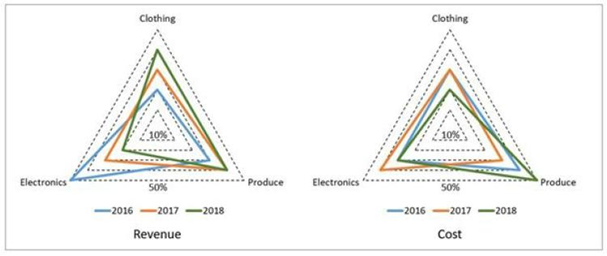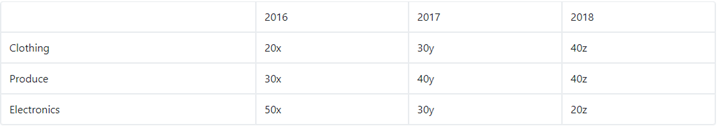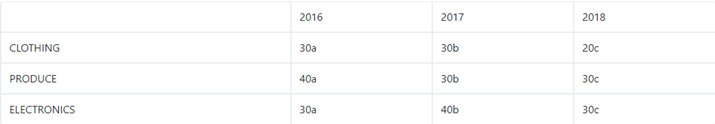### CAT 2019 - Slot 2 - Logical Reasoning and Data Interpretation - A large store has only three departments, Clothing, Produce, and Electronics

\$ 39
99
Monthly
• List Item #1
• List Item #2
Popular
##### A large store has only three departments, Clothing, Produce, and Electronics. The following figure shows the percentages of revenue and cost from the three departments for the years 2016, 2017 and 2018. The dotted lines depict percentage levels. So for example, in 2016, 50% of store's revenue came from its Electronics department while 40% of its costs were incurred in the Produce department.In this setup, Profit is computed as (Revenue – Cost) and Percentage Profit as Profit/Cost × 100%. It is known that 1. The percentage profit for the store in 2016 was 100%. 2. The store’s revenue doubled from 2016 to 2017, and its cost doubled from 2016 to 2018. 3. There was no profit from the Electronics department in 2017. 4. In 2018, the revenue from the Clothing department was the same as the cost incurred in the Produce department.

Q. 1: What was the percentage profit of the store in 2018?

##### Q. 2: What was the ratio of revenue generated from the Produce department in 2017 to that in 2018?1.  4 : 3 2. 16 : 93. 9 : 164. 8 : 5

Q. 3: : What percentage of the total profits for the store in 2016 was from the Electronics department?

Q. 4:What was the approximate difference in profit percentages of the store in 2017 and 2018?
1. 25.0
2. 33.3
3. 15.5
4. 8.3

1. 25

2. Option 4

3. 70

4. Option 4

Let the total revenue in 2016, 2017 and 2018 be 100x, 100y and 100z . The total cost in 2016, 2017 and 2018 be 100a, 100b and 100c.

The information given can be tabulated as below

REVENUECOSTAs revenue doubled in 2017 so 100y = 2(100x)

Or y = 2x

Also cost doubled in 2018 so 100c = 2(100a)

Or c = 2a

It is given that Profit in electronics in 2017 = 0

Which mean 30y = 40b

Percentage profit in 2016 = `100 -> x = 2a

In2018… -> 40z = 50c

Thus z =2.5a, x = 2a, y = 4a, b = 3a, c = 2a

profit in 2018 = (250a – 200a)/200a x 100 = 25

Rev produce in 2017/rev produce in 2018 = 40y/40z = 8/5

profit in 2016 = 100a. Profit from produce = 70a -> 70%

profit in 2017 = 400a – 300a / 300a = 33.33%

Profit in 2018 = 25%

Difference = 8.3

Inspiring Education… Assuring Success!!

Ghatkopar | Borivali | Andheri | Online